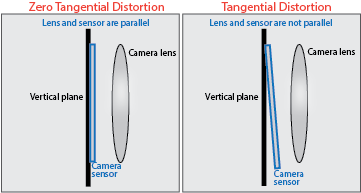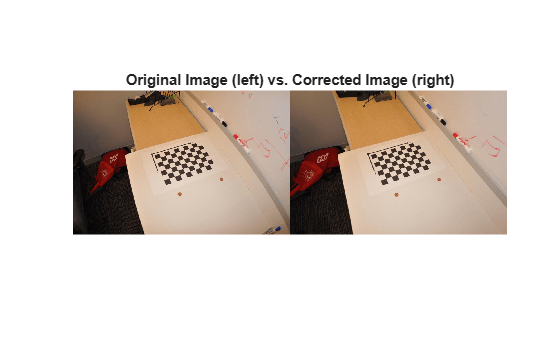# cameraParameters

Object for storing camera parameters

## Description

The `cameraParameters` object stores the intrinsic, extrinsic, and lens distortion parameters of a camera.

## Creation

You can create a `cameraParameters` object using the `cameraParameters` function described here. You can also create a `cameraParameters` object by using the `estimateCameraParameters` with an M-by-2-by-numImages array of input image points. M is the number of keypoint coordinates in each pattern.

### Syntax

``cameraParams = cameraParameters``
``cameraParams = cameraParameters(Name,Value)``
``cameraParams = cameraParameters(paramStruct)``

### Description

````cameraParams = cameraParameters` creates a `cameraParameters` object that contains the intrinsic, extrinsic, and lens distortion parameters of a camera.```

example

````cameraParams = cameraParameters(Name,Value)` sets properties of the `cameraParameters` object by using one or more name-value arguments. Unspecified properties use default values.For example, ```cameraParams = cameraParameters("RadialDistortion",[0 10])``` sets the radial lens distortion property, `RadialDistortion`, as the vector ```[0 10]```.```
````cameraParams = cameraParameters(paramStruct)` creates an identical `cameraParameters` object from an existing `cameraParameters` object with parameters stored in `paramStruct`.```

### Input Arguments

expand all

Camera parameters, specified as a camera parameters structure. To get a `paramStruct` from an existing `cameraParameters` object, use the `toStruct` function.

expand all

## Intrinsic Camera Parameters:

Camera intrinsic matrix, specified as a 3-by-3 matrix. The matrix has this format:

`$\left[\begin{array}{ccc}{f}_{x}& s& {c}_{x}\\ 0& {f}_{y}& {c}_{y}\\ 0& 0& 1\end{array}\right]$`

The coordinates [cx cy] represent the optical center (the principal point), in pixels. When the x- and y-axes are exactly perpendicular, the skew parameter s equals `0`.

fx = F*sx

fy = F*sy

• F is the focal length in world units, typically expressed in millimeters.

• sx and sy are the number of pixels per world unit in the x- and y-direction respectively.

• fx and fy are expressed in pixels.

Camera intrinsics object, stated as a `cameraIntrinsics` object. The object contains information about camera intrinsic calibration parameters, including lens distortion.

#### Dependency

You must provide an image size (using the `ImageSize` property) for the `Intrinsics` property to be non-empty. The intrinsics for the camera parameters depends on the image size.

Image size, specified as a two-element vector [mrows ncols].

## Camera Lens Distortion:

Radial distortion coefficients, specified as either a two- or three-element vector. When you specify a two-element vector, the object sets the third element to `0`. Radial distortion is the displacement of image points along radial lines extending from the principal point.

The camera parameters object calculates the radial-distorted location of a point. You can denote the distorted points as (xdistorted, ydistorted), as follows:

xdistorted = x(1 + k1*r2 + k2*r4 + k3*r6)

ydistorted= y(1 + k1*r2 + k2*r4 + k3*r6)

 x, y are undistorted pixel locations k1, k2, and k3 are radial distortion coefficients of the lens r2 = x2 + y2
Typically, two coefficients are sufficient. For severe distortion, you can include k3. The undistorted pixel locations appear in normalized image coordinates, with the origin at the optical center. The coordinates are expressed in world units.

Tangential distortion coefficients, specified as a two-element vector. Tangential distortion occurs when the lens and the image plane are not parallel. The camera parameters object calculates the tangential distorted location of a point. You can denote the distorted points as (xdistorted, ydistorted). The undistorted pixel locations appear in normalized image coordinates, with the origin at the optical center. The coordinates are expressed in world units.

Tangential distortion occurs when the lens and the image plane are not parallel. The tangential distortion coefficients model this type of distortion.The distorted points are denoted as (xdistorted, ydistorted):

xdistorted = x + [2 * p1 * x * y + p2 * (r2 + 2 * x2)]

ydistorted = y + [p1 * (r2 + 2 *y2) + 2 * p2 * x * y]

• x, y — Undistorted pixel locations. x and y are in normalized image coordinates. Normalized image coordinates are calculated from pixel coordinates by translating to the optical center and dividing by the focal length in pixels. Thus, x and y are dimensionless.

• p1 and p2 — Tangential distortion coefficients of the lens.

• r2 = x2 + y2

## Extrinsic Camera Parameters:

Calibration pattern extrinsics, specified as a P-element vector of `rigidtform3d` objects. Each object stores information about the 3-D rotation matrices and the camera translation vectors.

• The `R` property of each `rigidtform3d` object describes the 3-D rotation of the camera image plane relative to the corresponding calibration pattern.

• The `Translation` property of each `rigidtform3d` object describes the translation t of the camera relative to the corresponding calibration pattern, expressed in world units.

This equation provides the transformation that relates a world coordinate in the checkerboard frame [X Y Z] and the corresponding image point [x y]:

`$w\left[\begin{array}{c}x\\ y\\ 1\end{array}\right]=K\left[\begin{array}{cc}R& t\end{array}\right]\left[\begin{array}{c}X\\ Y\\ Z\\ 1\end{array}\right]$`

• w: arbitrary scale factor

• K: camera intrinsic matrix

• R: matrix representing the 3-D rotation of the camera

• t: translation of the camera relative to the world coordinate system

The rigid geometric transformations do not take distortion into consideration. Use the `undistortImage` function to remove distortion.

3-D rotation vectors, specified as a P-by-3 matrix containing P rotation vectors. Each vector describes the 3-D rotation of the camera image plane relative to the corresponding calibration pattern. The vector specifies the 3-D axis about which the camera is rotated, where the magnitude is the rotation angle in radians. The `PatternExtrinsics` property specifies geometric transformation objects with the corresponding 3-D rotation matrices.

## Estimated Camera Parameter Accuracy:

Average Euclidean distance between reprojected and detected points, specified as a numeric value in pixels.

Estimated camera parameters accuracy, specified as an M-by-2-by-P array of [x y] coordinates. The [x y] coordinates represent the translation in x and y between the reprojected pattern key points and the detected pattern key points. The values of this property represent the accuracy of the estimated camera parameters. P is the number of pattern images that estimates camera parameters. M is the number of keypoints in each image.

World points reprojected onto calibration images, specified as an M-by-2-by-P array of [x y] coordinates. P is the number of pattern images and M is the number of keypoints in each image. Missing points in the pattern's detected keypoints are denoted as [`NaN,NaN`].

Detected keypoints in the calibration pattern, specified as a logical M-by-P array. M is the number of keypoints in the entire calibration pattern and P specifies the number of calibration images.

## Settings for Camera Parameter Estimation:

Number of calibration patterns that estimates camera extrinsics, specified as an integer. The number of calibration patterns equals the number of translation and rotation vectors.

World coordinates of key points on calibration pattern, specified as an M-by-2 array. M represents the number of key points in the pattern.

World points units, specified as a character vector or string scalar. The value describes the units of measure.

Estimate skew flag, specified as a logical scalar. When you set the logical to `true`, the object estimates the image axes skew. When you set the logical to `false`, the image axes are exactly perpendicular.

Number of radial distortion coefficients, specified as the number `2` or `3`.

Estimate tangential distortion flag, specified as the logical scalar `true` or `false`. When you set the logical to `true`, the object estimates the tangential distortion. When you set the logical to `false`, the tangential distortion is negligible.

## Examples

collapse all

Use the camera calibration functions to remove distortion from an image. This example creates a `cameraParameters` object manually, but in practice, you would use the `estimateCameraParameters` or the Camera Calibrator app to derive the object.

Create a `cameraParameters` object manually.

```k = [715.2699 0 565.6995; 0 711.5281 355.3466; 0 0 1]; radialDistortion = [-0.3361 0.0921]; cameraParams = cameraParameters("K",k,"RadialDistortion",radialDistortion)```
```cameraParams = cameraParameters with properties: Camera Intrinsics Intrinsics: [0x0 cameraIntrinsics] Camera Extrinsics PatternExtrinsics: [0x1 rigidtform3d] Accuracy of Estimation MeanReprojectionError: NaN ReprojectionErrors: [0x2 double] ReprojectedPoints: [0x2x0 double] Calibration Settings NumPatterns: 0 DetectedKeypoints: [0x2 double] WorldPoints: [0x2 double] WorldUnits: 'mm' EstimateSkew: 0 NumRadialDistortionCoefficients: 2 EstimateTangentialDistortion: 0 ```

Remove distortion from the images.

```I = imread(fullfile(matlabroot,"toolbox","vision","visiondata","calibration","mono","image01.jpg")); J = undistortImage(I,cameraParams);```

Display the original and the undistorted images.

```montage({I,J}) title("Original Image (left) vs. Corrected Image (right)")```Zhang, Z. "A Flexible New Technique for Camera Calibration." IEEE Transactions on Pattern Analysis and Machine Intelligence 22, no. 11 (November 2000): 1330–34. https://doi.org/10.1109/34.888718.

 Heikkila, J., and O. Silven. “A Four-Step Camera Calibration Procedure with Implicit Image Correction.” In Proceedings of IEEE Computer Society Conference on Computer Vision and Pattern Recognition, 1106–12. San Juan, Puerto Rico: IEEE Comput. Soc, 1997. https://doi.org/10.1109/CVPR.1997.609468.